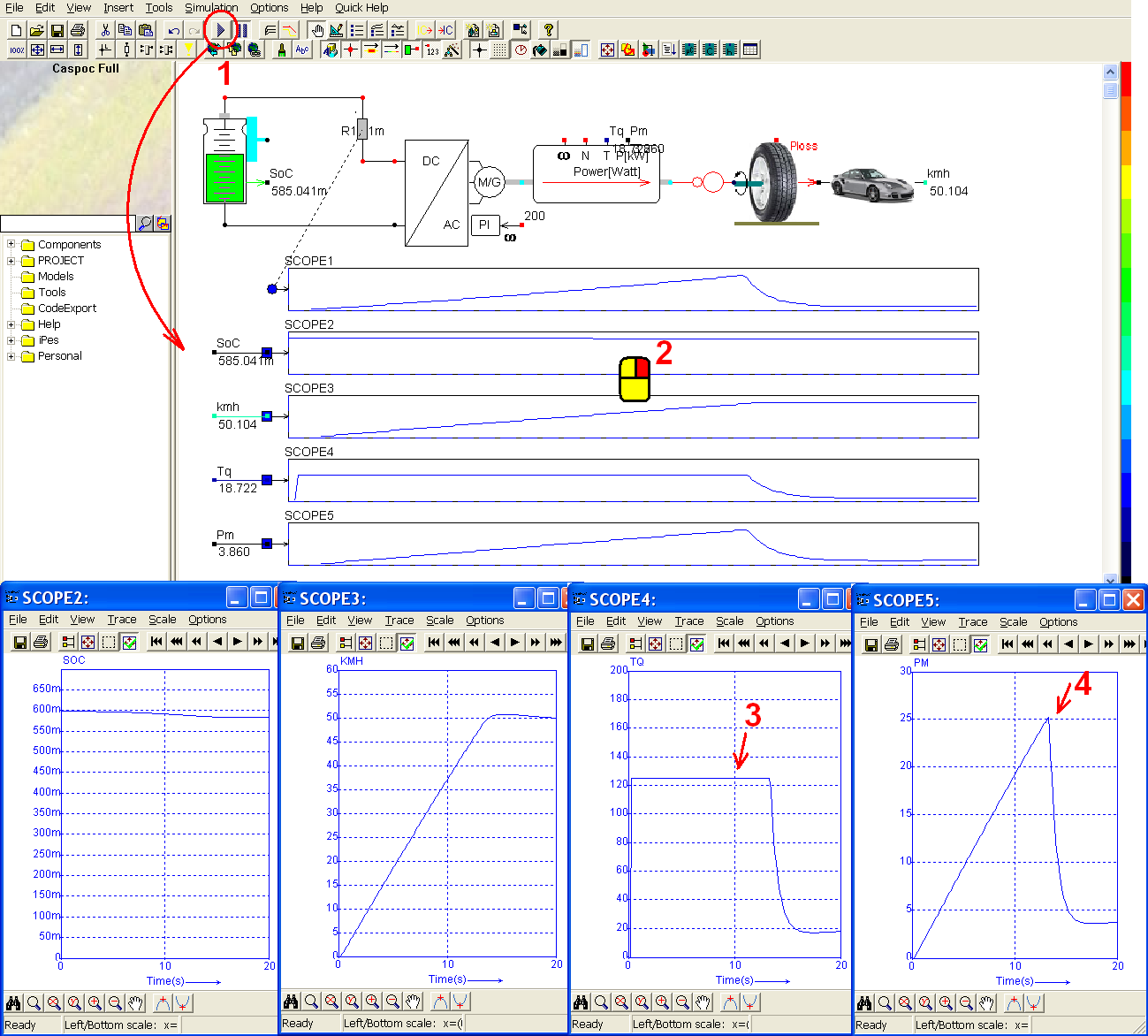• Introduction
• Power Electronics
• SMPS
• DC Drives
• AC Drives
• Drives
• Green Energy

## Electric Vehicle

An electric vehicle includes the power supply (battery and its DC circuit), DC/AC converter (convert the current from the battery to the motor), AC motor, main shaft, gear, wheel and the vehicle information. The model is shown as below. From the, we can see that the driver gives a desired angular speed to the DC/AC converter, and then the converter adapts its 3-phase current to the AC motor in order to control the angular speed of the main shaft. The smaller the angular speed of the main shaft, the smaller the car speed is. In other words, the driver can control the car speed by giving a desired angular speed to the converter. The battery will be discharged according the energy consumption of the control circuit and the motor. We can get the information of its state of charge which equals to 1 � state of discharge by using the SoC output of the battery.First, we build a DC circuit for the battery. Left-click Components/Library/Electric/Battery/LiIonModule (step 1) and release the mouse. The Li-Ion module follows the cursor and left-click on the workscreen to place it. Right-click on the Li-Ion module to modify its parameters (step 2). The parameters of the Li-Ion module is already explained in the previous simple example. Here we modify the initial SoC and the number of battery in parallel and in series connection (step 3):

• Nparallel = 7 (number of cells connected in parallel inside the battery module)
• Nseries = 200 (number of cells connected in series inside the battery module)
• SoC (time=0) = 60 %

Notices that all the parameters in other fields are specified for only a single cell.

Click OK to save the setting (step 4).Right-click on the second output of the battery (step 1) to add a label. In the pop-window, enter label = SoC (step 2) and click OK to save the setting (step 3). The give a small resistance to the DC circuit. Left-click Components/Circuit/RLC/R (step 4) and release the mouse. The resistor follows the cursor and right-click to change its direction (step 5). Until the anode of the resistor is upward, left-click on the workscreen to put the resistor in the right side of the battery. Right-click the resistor (step 6) to modify its parameter. In the pop-window, enter value = 1m (ohm) (step 7) and click OK to save the setting (step 8).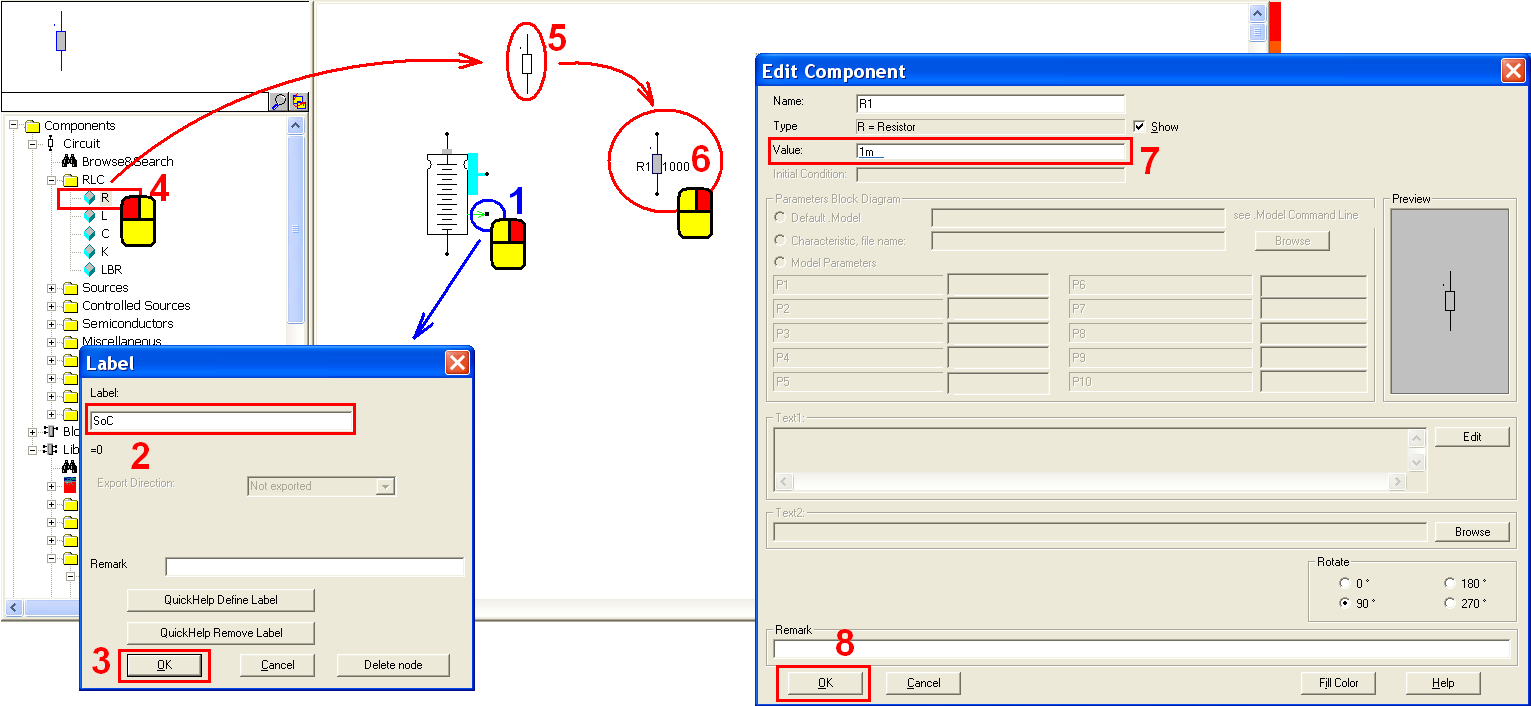Take the drive which includes the DC/AC converter and the motor. Left-click Components/Library/ElectricalMachine/System/VariableSpeedDriveControlled (step 1) and release the mouse. Left-click on the workscreen to put the drive in the right side of the battery (step 2). Right-click on the drive and modify its parameters. In the pop-window, we can see the parameters as below:

• Constant Power Speed Range [from 1 to 10] = 3 (how fast the drive can rotate and still produce power). Here we set this parameter to 3. It means that the drive can rotate up to 3* rpm_nominal rotating/minute.
• Efficiency [from 0 to 1] = 0.95 (the efficiency of the drive from DC input to mechanical output)
• Inertia = 100m kgm� (inertia of the shaft)
• K = 10 (one of the PI control parameters for the proportional part)
• Pmax = 40k Watts (the maximum power of the entire drive system which is 40kW for a very small car, 80kW for a normal size of 4-person family, and up to 120-150kW for a Mercedes Benz or BMW)
• Vdc Minimum = 500 (the minimum DC voltage to drive the drive) (the drive will stop while the DC voltage is below Vdc minimum)
• rpm_nominal = 3000 (nominal rpm). The maximum torque can be delivered by the drive up to the nominal rpm. While the rotating speed is above the nominal rpm, the delivered torque will decrease according to Pmax/ω (angular speed).
• rpm_nominal = 3000 (nominal rpm). The maximum torque can be delivered by the drive up to the nominal rpm. While the rotating speed is above the nominal rpm, the delivered torque will decrease according to Pmax/ω (angular speed).
• Tau = 10 (one of the PI control parameters for the integral part)

From the parameters, we can know the maximum torque = Pmax / ω = Pmax / (rpm_nomial * 2 * π /60 ) = 40k / (3000 * 2π / 60) = 127.32. Hence the maximum torque will be always lower than 127.32 considering the maximum power Pmax.

Here the parameter constant power speed range is set to 3, it means that the drive can rotate up to 3* rpm_nominal = 3 * 3000. Then torque = Pmax / ω = Pmax / (3* rpm_nomial * 2 * π / 60) = 40k / (3 * 3000 * 2π /60) = 42.44 (the rotating speed increases so that the torque decreases).

Click OK to save the setting (step 4).Connect the battery, the resistor and the drive with the following configuration (step 1). Right-click on ω input to give a requested angular speed (step 2). In the pop-window, enter label = 200 (step 3) and click OK to save the setting (step 4).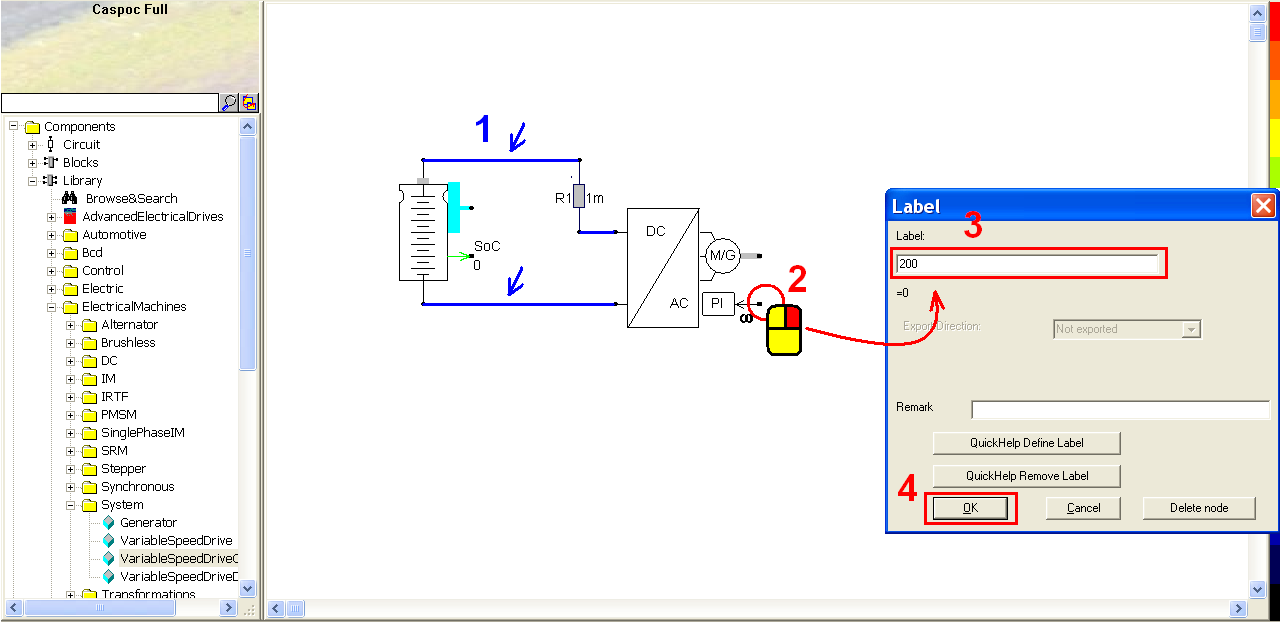Before connecting to the mechanical load, add a power torque sensor on the shaft. Left-click Components/Library/Sensor/Rotational/PowerTorque (step 1) and release the mouse. Right-click on the workscreen to put the power torque sensor in the right side of the drive (step 2). Make sure the input of the power torque sensor is well connected to the shaft of the motor (step 3). Then add labels for the torque and the power outputs. Right-click on the torque output (step 4) to add a label Tq (torque). In the pop-window, enter label = Tq (step 5) and click OK to save the setting (step 6). Right-click on the power output (step 7) to add a label Pm (mechanical power). In the pop-window, enter label = Pm (step 8) and click OK to save the setting (step 9).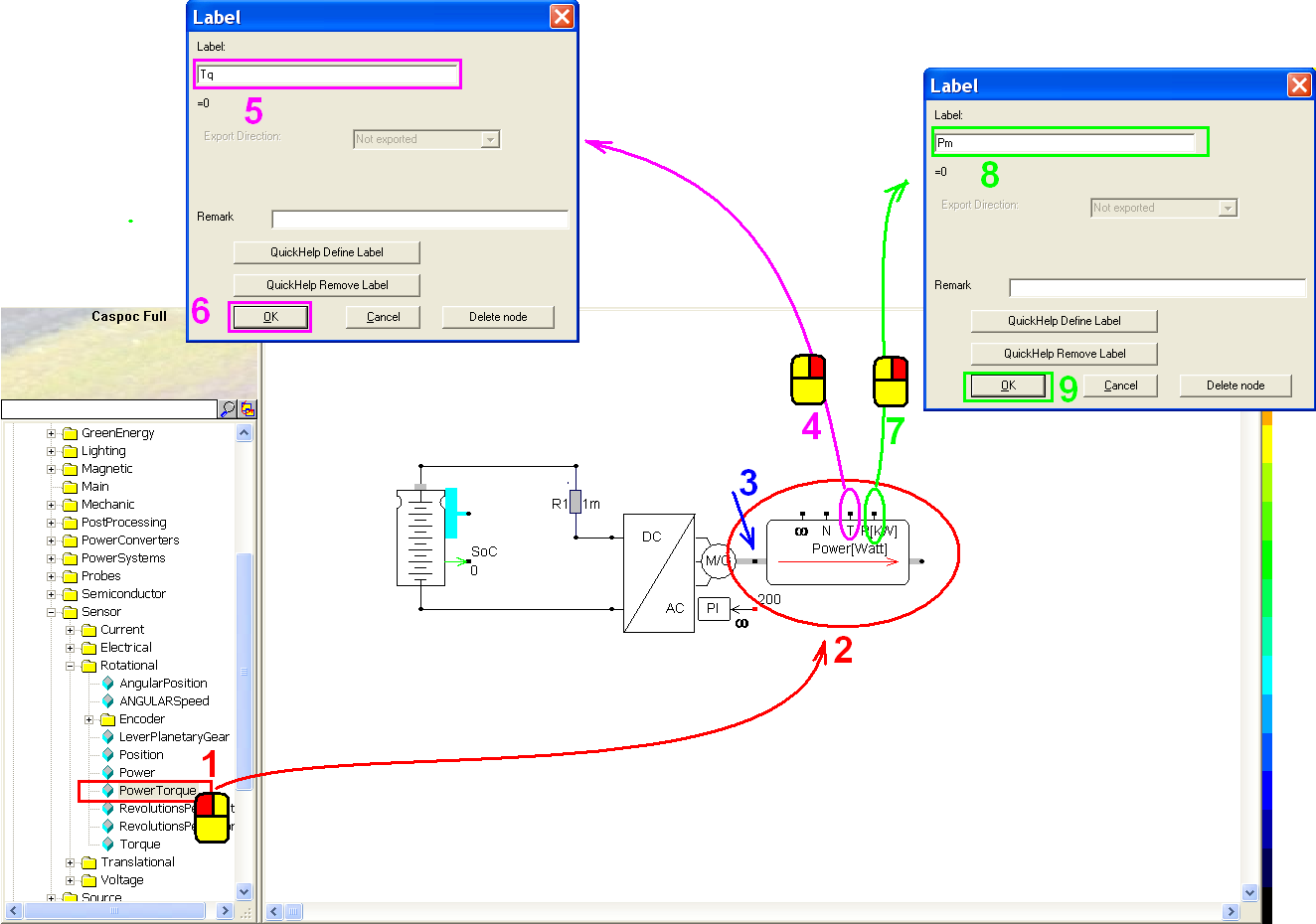Then we use a gear to change the angular speed and torque according to their teeth number. After the transmission, the product of the torque and the angular speed still equals to the mechanical power. Left-click Components/Library/Mechanic/Rotational/Gear (step 1) and release the mouse. Left-click on the workscreen to put the gear in the right side of the power torque sensor. Make sure that gear is well connected with the power torque sensor (step 2). Right-click the gear to modify its parameters (step 3). In the pop-window, we can see the parameters as below (step 4):

• Efficiency [from 0 to 1] = 0.98 (the efficiency of the gear which affects the power losses)
• NumberPrimaryTeeth = 1 (the number of teeth for the primary gear)
• NumberSecondaryTeeth = 4 (the number of teeth for the secondary gear)

Click OK to save the setting (step 5).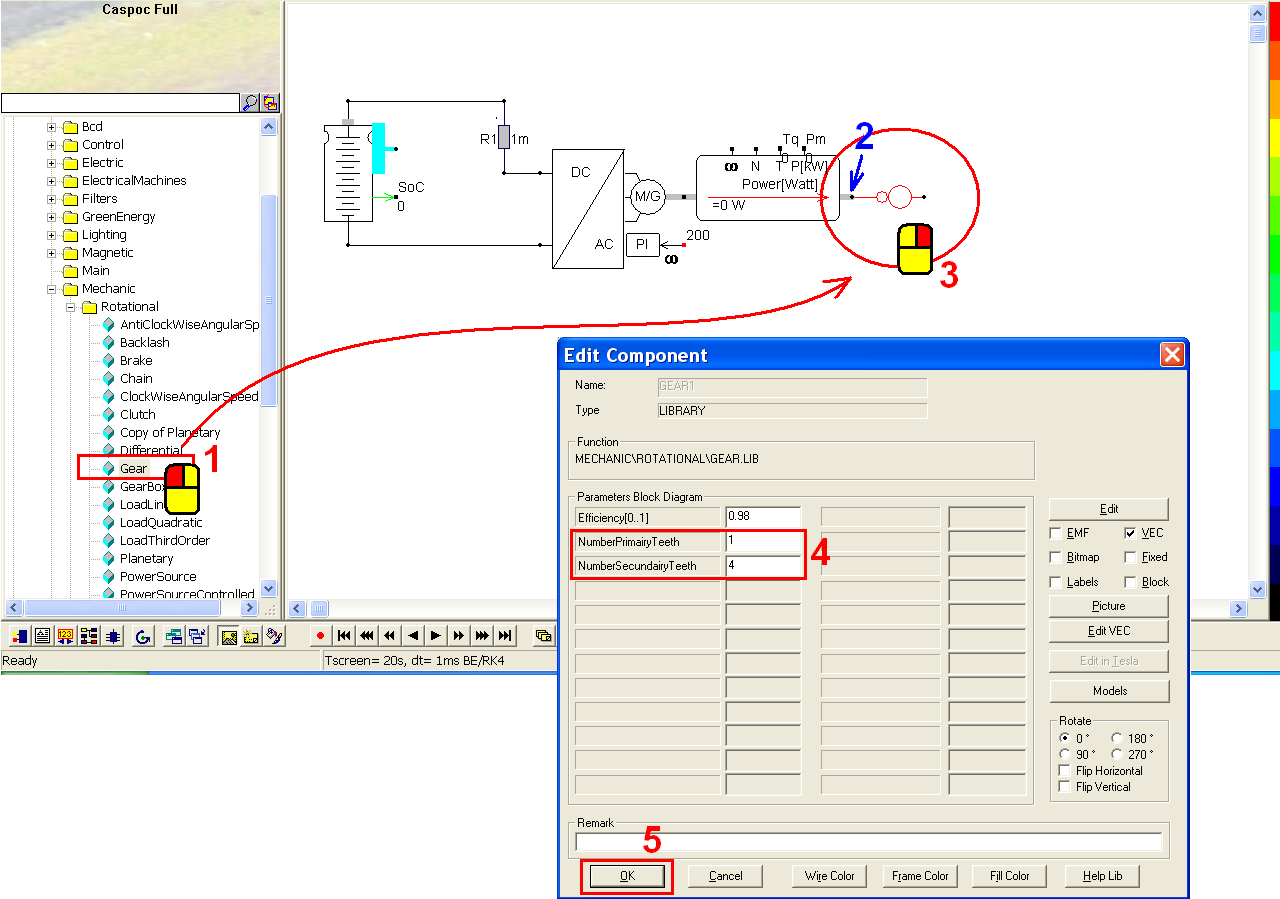Set the wheel slip for the vehicle. Left-click Components/Library/Mechanic/Transformation/WheelSlip (step 1) and release the mouse. Left-click on the workscreen to put the wheel in the right side of the gear. Make sure that the wheel is well connected with the gear (step 2). Right-click on the wheel to modify its parameters (step 3). In the pop-window, we can see the parameters as below (step 4):

• R = 0.3 meters (wheel radius)
• Slip [from 0 to 1] = 0.1 (slip between the tire and road)

Click OK to save the setting (step 5).Give the information of a vehicle. Left-click Components/Library/Mechanic/Translational/CarRollingDragResistance (step 1) and release the mouse. Left-click on the workscreen put the vehicle in the right side of the wheel. Make sure that the vehicle is well connected with the wheel (step 2). Right-click on the vehicle (step 3) to modify its parameters. In the pop-window, we can see the parameters as below (step 4):

• Air Density = 1 kg/m� (the average value of air density)
• Car Mass = 1400 kg (total mass of the car)
• Cr(RollingCoefficient) = 0.01 N/kg (rolling coefficient of the wheel)
• Cw(DragCoefficient) = 0.4 (drag coefficient around 0.3 to 0.4)
• Frontal Area = 2.2 m� (frontal area of the car)

Click OK to save the setting (step 5).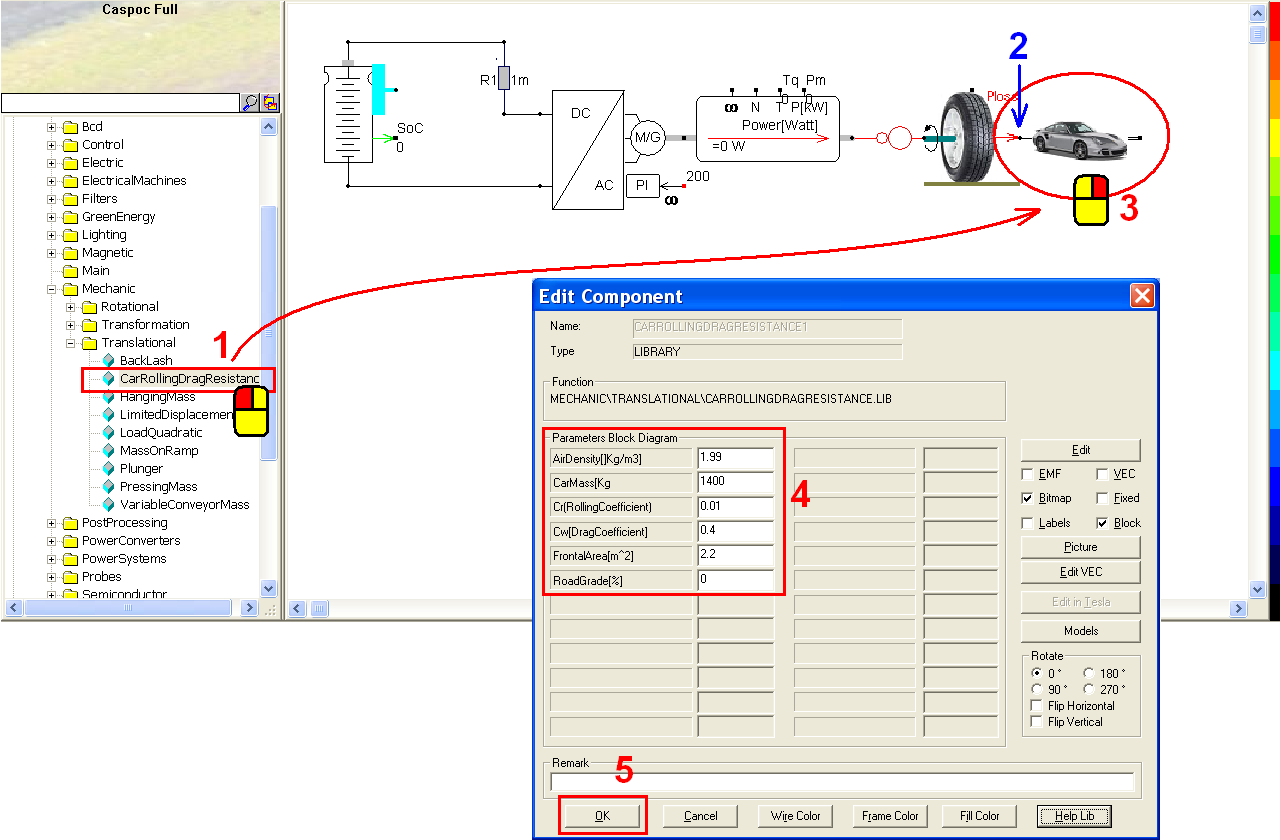Right-click the speed output of the vehicle (step 1) to add a label. In the pop-window, enter label = kmh (step 2) and click OK to save the setting (step 3).Take five scope for reading five different information. Click the scope iconin the experience bar (step 1) to put the first scope on the workscreen. Left-click the right-bottom corner of the scope and hold down the mouse to enlarge this scope (step 2). Repeat the same steps to put the second, third, fourth and fifth scopes (step 3~10).Use first scope to measure the current through the resistor (step 1). Right-click the blue input of the first scope (step 2). In the pop-window, enter Trace=Current through: R1 (step 3) and click OK to save the setting (step 4).Extend the first blue input of the second, third, fourth and fifth scopes. Left-click the blue round dot, draw the mouse and then release the mouse (step 1). Then we can see the blue round dot becomes a square dot. Repeat the same steps with the third, fourth and fifth scopes (step 2, 3 and 4). Then we will use the four scopes to read the labels SoC, kmh, Tq and Pm which are marked in green.Right-click the input of second scope (step 1). In the pop-window, enter label = SoC (step 2) and click OK to save the setting (step 3).

Right-click the input of third scope (step 4). In the pop-window, enter label = kmh (step 5) and click OK to save the setting (step 6).

Right-click the input of fourth scope (step 7). In the pop-window, enter label = Tq (step 8) and click OK to save the setting (step 9).

Right-click the input of fifth scope (step 10). In the pop-window, enter label = Pm (step 11) and click OK to save the setting (step 12).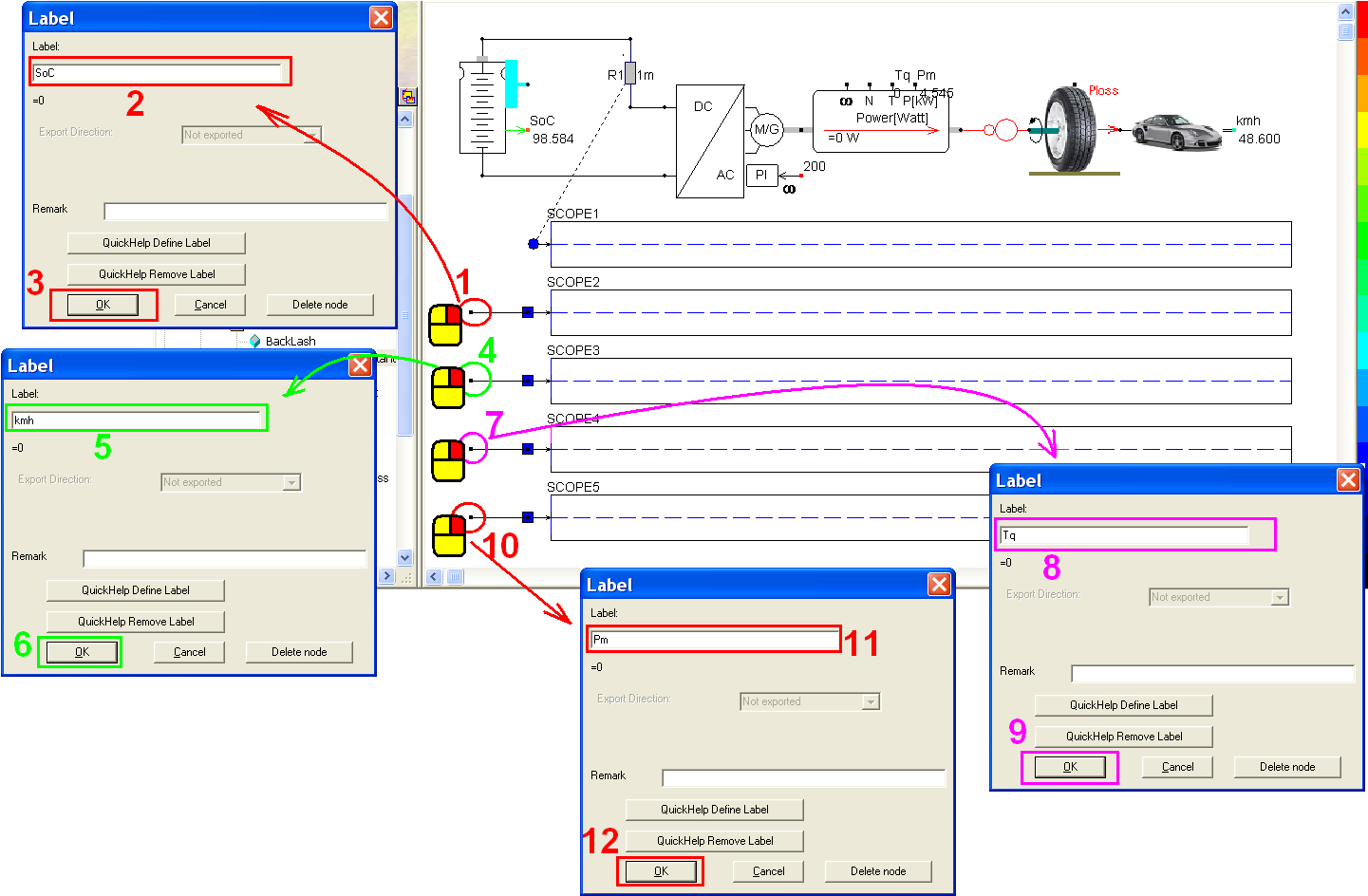Click the short-cut of simulation parameter(step 1). In the pop-window, select Euler for the Numerical Integration Method (step 2), Tscreen = 20, dt = 100m (step 3) and then click OK to save the setting (step 4).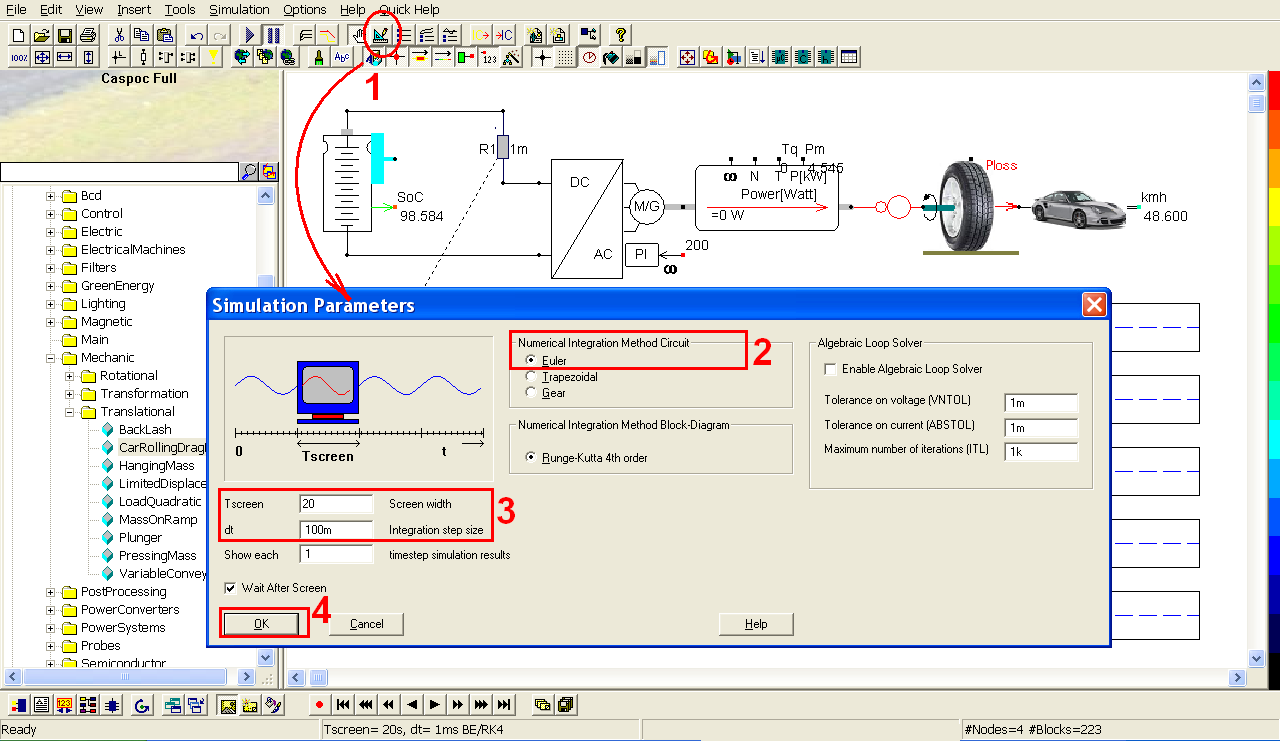Click the short-cut of start simulation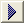(step 1). Right-click on these scopes (step 2) to see the details of the simulation result.

The second scope shows the decrease of the SoC from 60% (according to our settings in the battery).

The third scope shows the car speed which increases in the beginning and then keep about 50 km/h after 20 seconds.

The fourth scope shows the torque of the shaft. As mentioned previously, the maximum torque is up to 127.32 so that we can see the drive tried to reach the requested angular speed 200 rad/s by holding the maximum torque in the beginning (step 3). Once the requested angular speed is reached, the torque decreases very fast.

The fifth scope shows the power measured from the shaft. The power equals to the product of the torque and the angular speed. From fourth scope, we know the maximum torque is about 125 so that the maximum power equals 125 * 200 = 25kW (step 4).